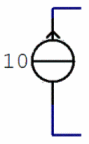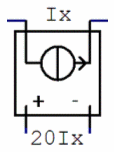Simple Circuit – Analysis with Matlab

In the following exercise we are going to analyze a simple circuit with Matlab. This is the logic next step or a continuation of the nodal analysis example that we previously worked on the page dedicated to Nodal Analysis using the KCL.

 This circuit contains more components: 6 resistors (in ohms), a current source (in amperes), a voltage source (in volts) and a voltage source that produces its voltage depending on a current going through another branch of the circuit (that’s called a current-controlled voltage source, or CCVS).

The schematic for our example is as follows:This is the symbol of the constant current source:In particular, the current through this source is 10 A.

This is the symbol of the current-controlled voltage source:In particular, this source is creating a voltage of 20 times the current Ix, that goes through the top branch of the circuit, that is, through the 4-ohm resistor.

Our goal is to discover or calculate the values of the voltages on the shown four nodes, V1 to V4.

Proposed Solution

We are going to solve a linear system that represents our circuit. We have 4 variables and can study 4 nodes (the remaining node is the reference node or ground), and we are going to solve the linear equation YV = I by using the left division available in Matlab, V = Y\I, where Y is the square matrix (4 × 4) of coefficients of the unknowns in our system, V is the 4-element column vector representing the nodes, and I is the 4-element column vector of constants on the right of the system. Each row of our matrices is going to summarize the analysis of each node, while each column is going to contain the coefficients of the unknowns.

Kirchhoff’s current law (KCL) states that for any electrical circuit, the algebraic sum of all the currents at any node in the circuit equals zero.

Analysis

At node 1, we have
Current going to it, 10 A.
Currents going out from it, V1/40, (V1 - V2)/10, and (V1 - V4)/4

In mathematics that means:
10 = V1/40 + (V1 - V2)/10 + (V1 - V4)/4

Rearranging the above numbers, the first row of our Y matrix is:
Y(1, :) = [(1/40 + 1/4 + 1/10) (-1/10) 0 -1/4]
And the first element of our column I is:
I(1) = 10

At nodes 2 and 3, we can see that
V2 – V3 = 20Ix, but we also know that Ix = (V1 - V4)/4
that means that
V2 – V3 = 20(V1 - V4)/4

and we conclude that the second row of our Y matrix is:
Y(2, :) = [5 -1 1 -5]
The second element of our column I is
I(2) = 0

From supernodes 2 and 3, we get
(V2 – V1)/10 + V2/8 + V3/20 + (V3 - V4)/30 = 0

This produces our third row of the Y matrix:
Y(3, :) = [-1/10 (1/10 + 1/8) (1/20 + 1/30) -1/30]

The third element of I is
I(3) = 0

And at node 4, we easily see that V4 = 20.
This means that
Y(4, :) = [0 0 0 1]
and
I(4) = 20

Because of the above explanation, our Y matrix is
Y = [
(1/40 + 1/4 + 1/10) -1/10 0 -1/4
5  -1 1 -5
-1/10 (1/10 + 1/8) 1/20 + 1/30 -1/30
0 0 0 1]

Our constant vector on the right of our system is
I = [5 0 0 20]’

And, thanks to the left division, we can easily solve our simple circuit, by just typing
V = Y\I

Nodal voltages V1, V2, V3, V4 are
V  =
36.2215
35.8306
- 45.2769
20

From 'Simple Circuit' to home

From 'Simple Circuit' to 'Electrical Calculations'

 Top